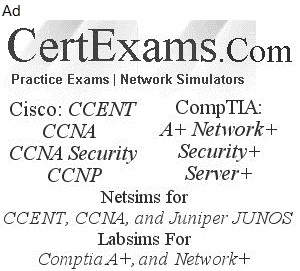# Juniper® JNCIA Exam Cram Notes : Binary Numbers To Decimal Number Conversion And Vice Versa

## 1. Networking Fundamentals

#### 1.7 Binary Numbers to Decimal Number Conversion and Vice Versa

Decimal is a Base 10 system with 10 possible values (0 to 9) and Binary is a Base 2 system with only two numbers 0 or 1.

i. Converting binary to decimal - The weightage of binary digits from right most bit position to the left most bit position is given below.

 27 26 25 24 23 22 21 20 128 64 32 16 8 4 2 1

Example: Convert 10011101 into a decimal value.

There are eight bits in the binary number. The decimal value for each bit position is given below:

 128 64 32 16 8 4 2 1 « Decimal equivalent of the binary position 1 0 0 1 1 1 0 1 « Given binary number

To convert, you simply take a value from the top row wherever there is a 1 below, and then add the values together. For instance, in our example we would have

1*27 + 0*26 + 0*25 + 1*24 + 1*23 + 1*22 + 0*21 + 1*20
= 128 + 0 + 0 + 16 + 8 + 4 + 0+ 1
= 157 (decimal value)

ii. Converting decimal to binary

To convert decimal to binary is also very simple, you simply divide the decimal value by 2 and then write down the remainder, repeat this process until you cannot divide by 2 anymore.

For example, take the decimal value 157:

157 ÷ 2 = 78 with a remainder of 1
78 ÷ 2 = 39 with a remainder of 0
39 ÷ 2 = 19 with a remainder of 1
19 ÷ 2 = 9 with a remainder of 1
9 ÷ 2 = 4 with a remainder of 1
4 ÷ 2 = 2 with a remainder of 0
2 ÷ 2 = 1 with a remainder of 0
1 ÷ 2 = 0 with a remainder of 1 <--- to convert, write this remainder first

Next write down the value of the remainders from bottom to top (in other words write down the bottom remainder first and work your way up the list) which gives: 10011101 = 157

Example: What is the possible decimal equivalent of 10101010?

 Position of Bit 7th 6th 5th 4th 3rd 2nd 1st 0th Decimal Value 128 64 32 16 8 4 2 1

Calculate the decimal equivalent based on the above predefined values.

Given Binary Number is: 10101010, it's decimal equivalent is:

1*(2^7) + 0*(2^6) + 1*(2^5) + 0*(2^4) + 1*(2^3) + 0*(2^2) + 1*(2^1) + 0*(2^0)=170 in decimal format.

JNCIA Junos Cram Notes Contents
• 1. Networking Fundamentals
• 2. Junos OS Fundamentals
• 3. User Interfaces
• 4. Junos Configuration Basics
• 5. Operational Monitoring and Maintenance
• 6. Routing Fundamentals
• 7. Routing Policy and Firewall Filters